# The Hardy-Weinberg EquilibriumPage 1

#### WATCH ALL SLIDES

Slide 1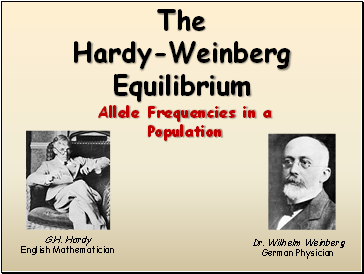The Hardy-Weinberg Equilibrium

Allele Frequencies in a Population

G.H. Hardy

English Mathematician

Dr. Wilhelm Weinberg

German Physician

Slide 2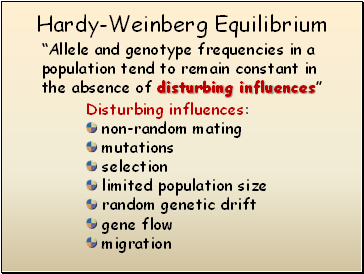## Hardy-Weinberg Equilibrium

“Allele and genotype frequencies in a population tend to remain constant in the absence of disturbing influences”

Disturbing influences:

non-random mating

mutations

selection

limited population size

random genetic drift

gene flow

migration

Slide 3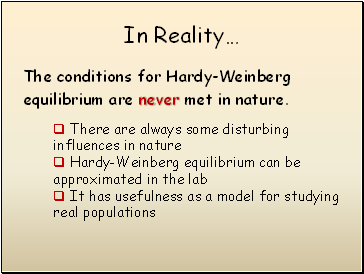## In Reality…

The conditions for Hardy-Weinberg

equilibrium are never met in nature.

There are always some disturbing influences in nature

Hardy-Weinberg equilibrium can be approximated in the lab

It has usefulness as a model for studying real populations

Slide 4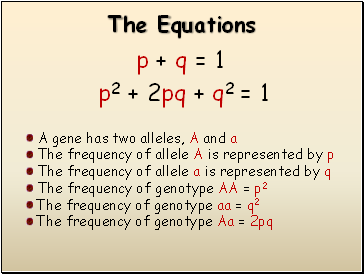## The Equations

p2 + 2pq + q2 = 1

A gene has two alleles, A and a

The frequency of allele A is represented by p

The frequency of allele a is represented by q

The frequency of genotype AA = p2

The frequency of genotype aa = q2

The frequency of genotype Aa = 2pq

p + q = 1

Slide 5## An Example…

Assume a population in which 36% of the population are homozygous for a certain recessive allele, a. Assume the population is at equilibrium.

Question #1: What is the frequency of the recessive allele, a in this population?

Slide 6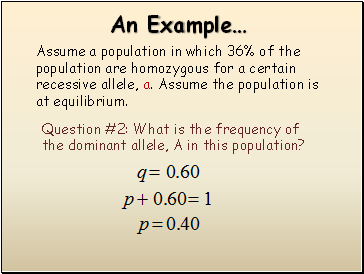An Example…

Assume a population in which 36% of the population are homozygous for a certain recessive allele, a. Assume the population is at equilibrium.

Question #2: What is the frequency of the dominant allele, A in this population?

Slide 7An Example…

Assume a population in which 36% of the population are homozygous for a certain recessive allele, a. Assume the population is at equilibrium.

Question #3: What percentage of the population are homozygous for the dominant allele, A?

Slide 8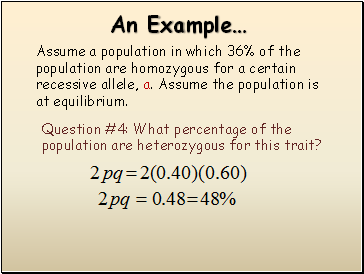An Example…

Assume a population in which 36% of the population are homozygous for a certain recessive allele, a. Assume the population is at equilibrium.

Question #4: What percentage of the population are heterozygous for this trait?

Slide 9An Example…

Assume a population in which 36% of the population are homozygous for a certain recessive allele, a. Assume the population is at equilibrium.

Go to page:
1  2